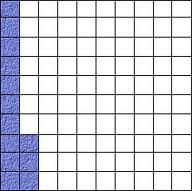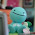## Monday, January 17, 2011

### Final Percent Post

The word percent means out of 100. Percents can be converted into decimals or fractions.
Ex. 65% - 65/100 - 0.65

Representing Percents 4.1

you can represent percents by using a hundred grid, since percents are out of 100.

Ex. this grid shows 13% since 13 squares are colored in.Fractions, Decimals, and Percents 4.2

Percents can be converted into fractions, or decimals.
Ex. 50% - 1/2 - 0.5

Percent of a number 4.3

To calculate the percent of a number, write the percent as a decimal and then multiply by the number.
Ex. 12 1/2 % of 50 = 0.125 x 50
=6.25

Combining Percents 4.4

Combine percents by adding to solve the problems.
Ex. 5% + 7% =12%

MY PERCENT VIDEO

#### 1 comment:

1.I like how you used different colours. I also like how you put a larger font for more noticeable things such as the header or the topic. You should add some italicized words to help people figure what is the more important parts of the post other than that, great job Ishaka!